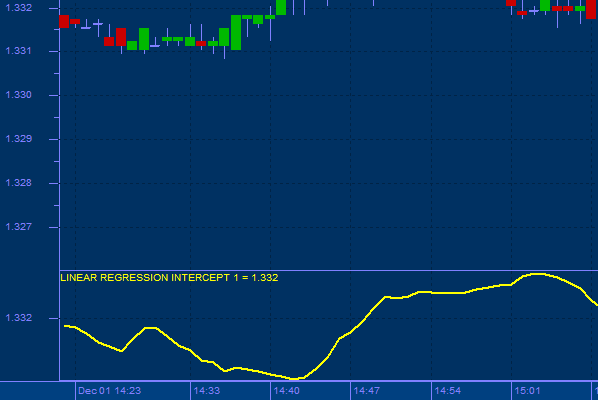top of page

## Linear Regression Intercept

A linear regression line is a straight line, which is as close to all of the given values as possible. The intercept component is the value of that line at bar #0.

Parameters

Data:  The data to use in the regression. This is typically a field in a data series or a calculated value.

Period: The number of bars of data to include in the regression, including the current value.

For example, a period of 3 includes the current value and the two previous values. The linear regression line at the beginning of a data series is not defined until there are enough values to fill the given period.

Use

The Linear Regression Intercept function determines the intercept to the first bar of the data series of a linear regression line of a field to time over a given number of periods.bottom of page